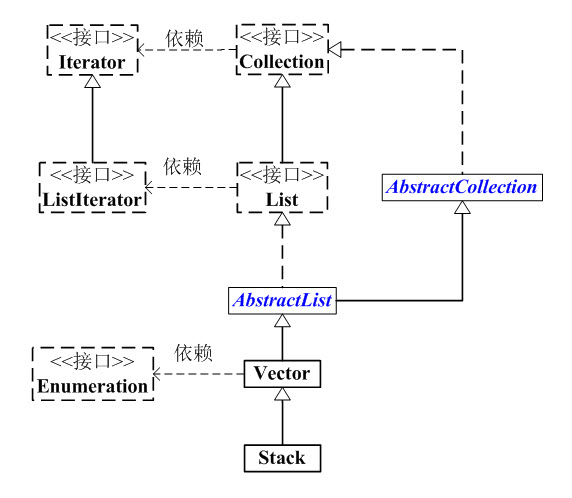# 第1部分 Stack介绍

## Stack简介

Stack是栈。它的特性是：先进后出(FILO, First In Last Out)。

Stack的继承关系

``````java.lang.Object
↳     java.util.AbstractCollection<E>
↳     java.util.AbstractList<E>
↳     java.util.Vector<E>
↳     java.util.Stack<E>
``````

Stack的声明

``````public class Stack<E> extends Vector<E> {}
``````

Stack和Collection的关系如下图：Stack的构造函数

``````Stack()
``````

Stack的API

``````         boolean       empty()
synchronized E             peek()
synchronized E             pop()
E             push(E object)
synchronized int           search(Object o)
``````

# 第2部分 Stack源码解析(基于JDK1.6.0_45)

Stack的源码非常简单，下面我们对它进行学习。

``````package java.util;

public
class Stack<E> extends Vector<E> {
// 版本ID。这个用于版本升级控制，这里不须理会！
private static final long serialVersionUID = 1224463164541339165L;

// 构造函数
public Stack() {
}

// push函数：将元素存入栈顶
public E push(E item) {
// 将元素存入栈顶。

return item;
}

// pop函数：返回栈顶元素，并将其从栈中删除
public synchronized E pop() {
E    obj;
int    len = size();

obj = peek();
// 删除栈顶元素，removeElementAt()的实现在Vector.java中
removeElementAt(len - 1);

return obj;
}

// peek函数：返回栈顶元素，不执行删除操作
public synchronized E peek() {
int    len = size();

if (len == 0)
throw new EmptyStackException();
// 返回栈顶元素，elementAt()具体实现在Vector.java中
return elementAt(len - 1);
}

// 栈是否为空
public boolean empty() {
return size() == 0;
}

// 查找“元素o”在栈中的位置：由栈底向栈顶方向数
public synchronized int search(Object o) {
// 获取元素索引，elementAt()具体实现在Vector.java中
int i = lastIndexOf(o);

if (i >= 0) {
return size() - i;
}
return -1;
}
}
``````

(01) Stack实际上也是通过数组去实现的。

(02) Stack继承于Vector，意味着Vector拥有的属性和功能，Stack都拥有。

# 第3部分 Vector示例

``````import java.util.Stack;
import java.util.Iterator;
import java.util.List;

/**
* @desc Stack的测试程序。测试常用API的用法
*
* @author skywang
*/
public class StackTest {

public static void main(String[] args) {
Stack stack = new Stack();
// 将1,2,3,4,5添加到栈中
for(int i=1; i<6; i++) {
stack.push(String.valueOf(i));
}

// 遍历并打印出该栈
iteratorThroughRandomAccess(stack) ;

// 查找“2”在栈中的位置，并输出
int pos = stack.search("2");
System.out.println("the postion of 2 is:"+pos);

// pup栈顶元素之后，遍历栈
stack.pop();
iteratorThroughRandomAccess(stack) ;

// peek栈顶元素之后，遍历栈
String val = (String)stack.peek();
System.out.println("peek:"+val);
iteratorThroughRandomAccess(stack) ;

// 通过Iterator去遍历Stack
iteratorThroughIterator(stack) ;
}

/**
* 通过快速访问遍历Stack
*/
public static void iteratorThroughRandomAccess(List list) {
String val = null;
for (int i=0; i<list.size(); i++) {
val = (String)list.get(i);
System.out.print(val+" ");
}
System.out.println();
}

/**
* 通过迭代器遍历Stack
*/
public static void iteratorThroughIterator(List list) {

String val = null;
for(Iterator iter = list.iterator(); iter.hasNext(); ) {
val = (String)iter.next();
System.out.print(val+" ");
}
System.out.println();
}

}
``````

``````1 2 3 4 5
the postion of 2 is:4
1 2 3 4
peek:4
1 2 3 4
1 2 3 4
``````